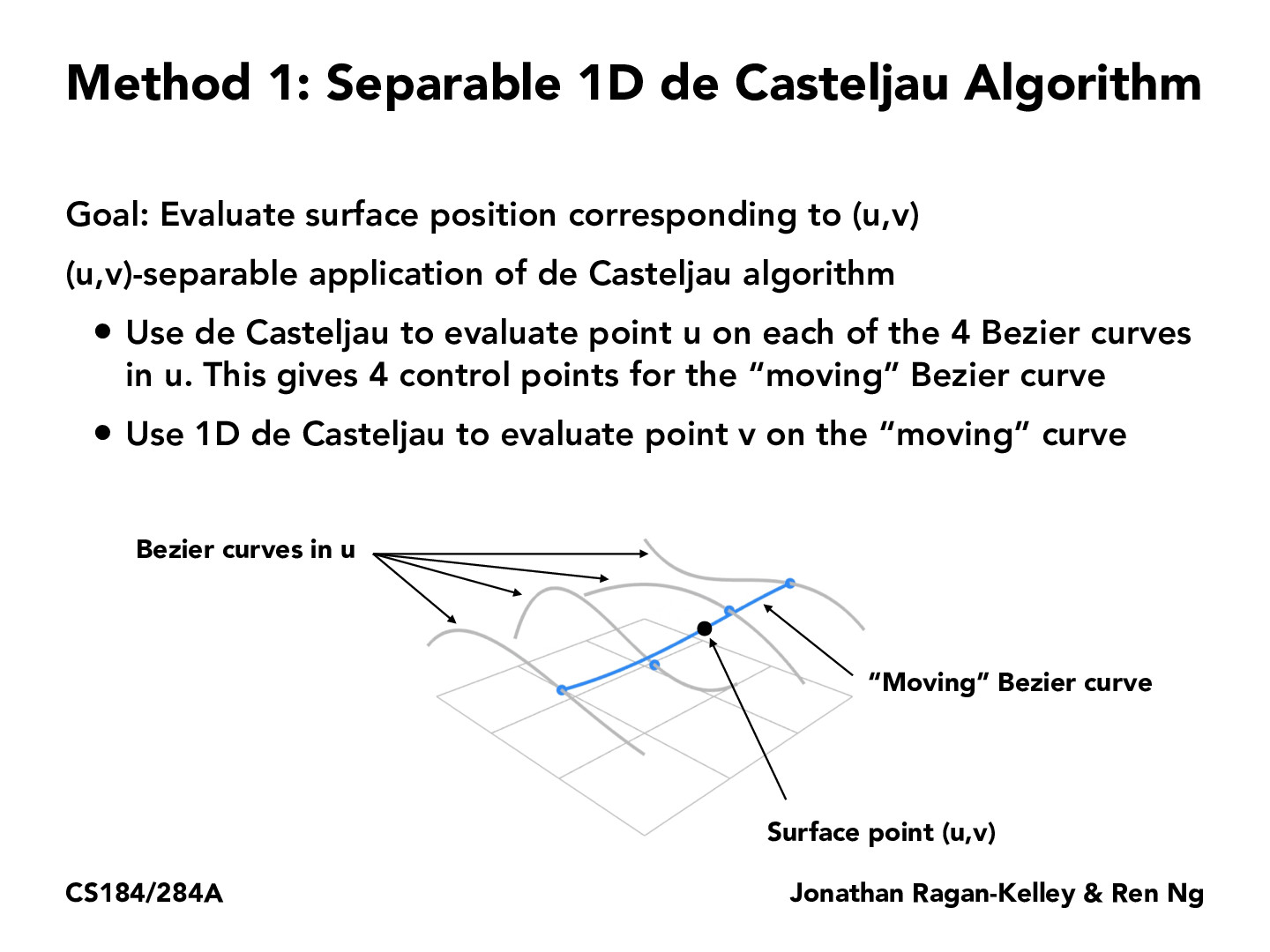You are viewing the course site for a past offering of this course. The current offering may be found here.Lecture 7: Geometry And Splines (90)jchen12197

I think I understand the de Casteljau Algorithm for curves where we get a point between two points using t as the constant ratio, create lines between the new points, and repeat until we are left with one point, but I don't really understand what "corresponding to (u,v)" means here for surfaces. Are u and v similar to what t does for single curves?theandrewchan

A 1-dimensional Bezier curve is parameterized by t in the way you describe. A 2-dimensional Bezier surface is defined over the uv-plane, and we can imagine taking a slice of the surface along the u-axis - this slice will be a one-dimensional Bezier curve parameterized by u. Similarly if we take a slice of the surface along the v-axis, the slice is equivalent to a one-dimensional Bezier curve parameterized by v. And where do we get these slices? For a 4x4 grid of control points, each row of points gives a slice along the u-axis and each column of points gives a slice along the v-axis!

You must be enrolled in the course to comment